1
AI & Data

# Day 38 - 在 AWS Lambda 中使用 YOLO 推估 (Inference)

Day 15 - 說明 YOLO 相關設定 以及 Day 16 - 進行影像辨識訓練完成了 YOLO 自訂資料集的訓練，在 Day 34 - 實作 S3 驅動 Lambda 函數進行 Yolo 物件辨識Day 36 - 使用 Container 建立 Amazon SageMaker 端點 分別用不同的方法來進行 YOLO 推估的任務。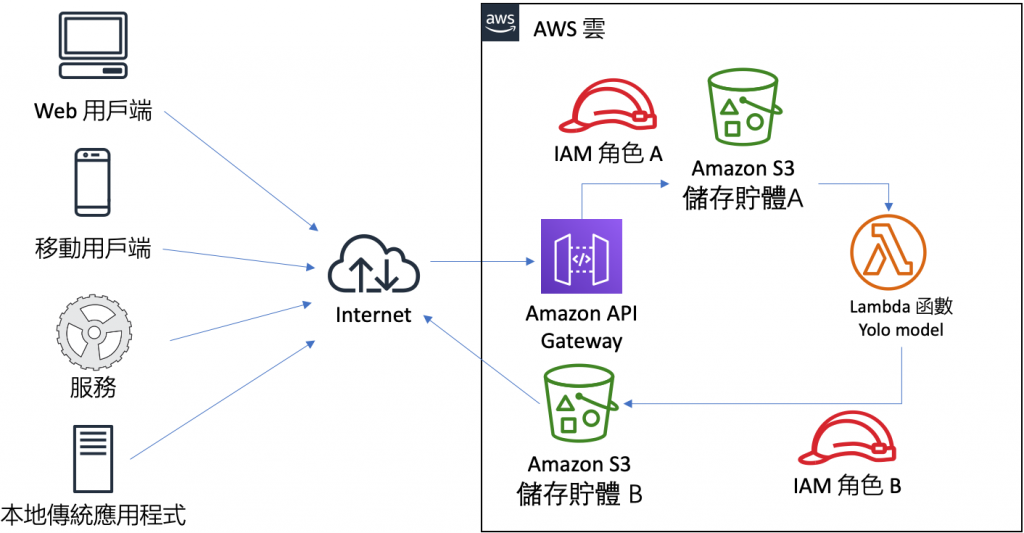Simple Inference Scripts for YOLO with OpenCV 這份專案中提供了一個使用 OpenCV 來進行 YOLO 推論的案例，現在我們就試著將這個案例佈署到 AWS Lambda 進行測試，需要的前期準備工作如下：

1. 上傳 YOLO 相關檔案到 S3： 訓練好的權重檔 (yolov3.backup) 以及待推論的圖片 (02-frame-608x608-0090.jpg)
2. 建立 IAM 角色，允許執行 AWS Lambda，並有存取 S3 的許可。
3. 建立 AWS Lambda 函數，並設定環境變數。
4. 在 AWS Lambda 建立 OpenCV 層
5. 撰寫 AWS Lambda 函數並完成測試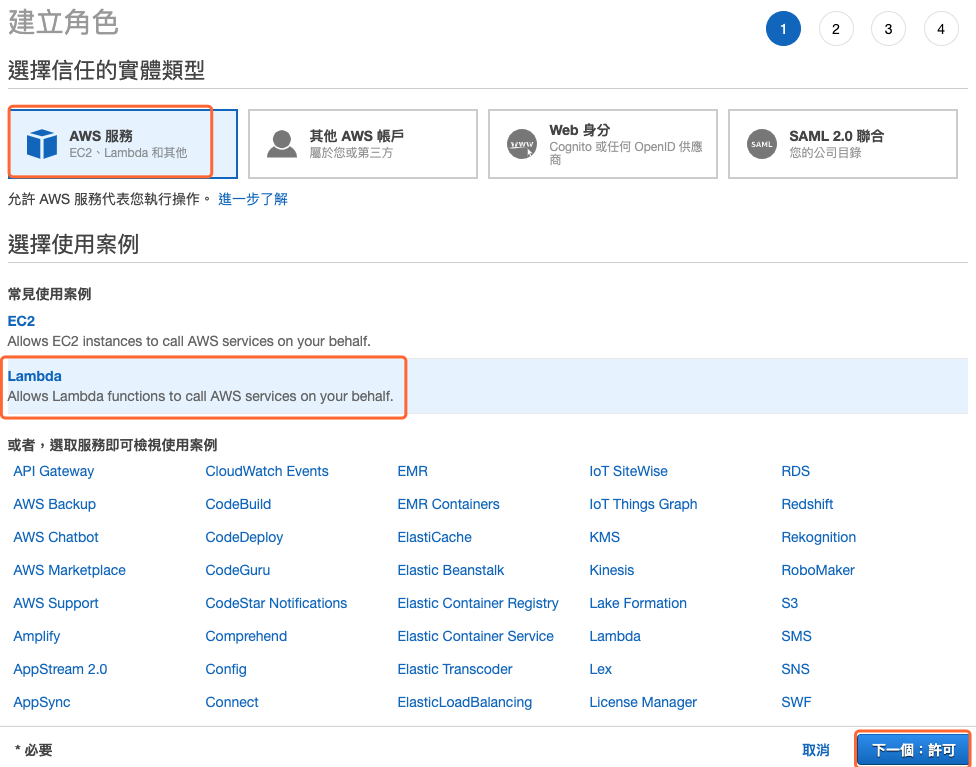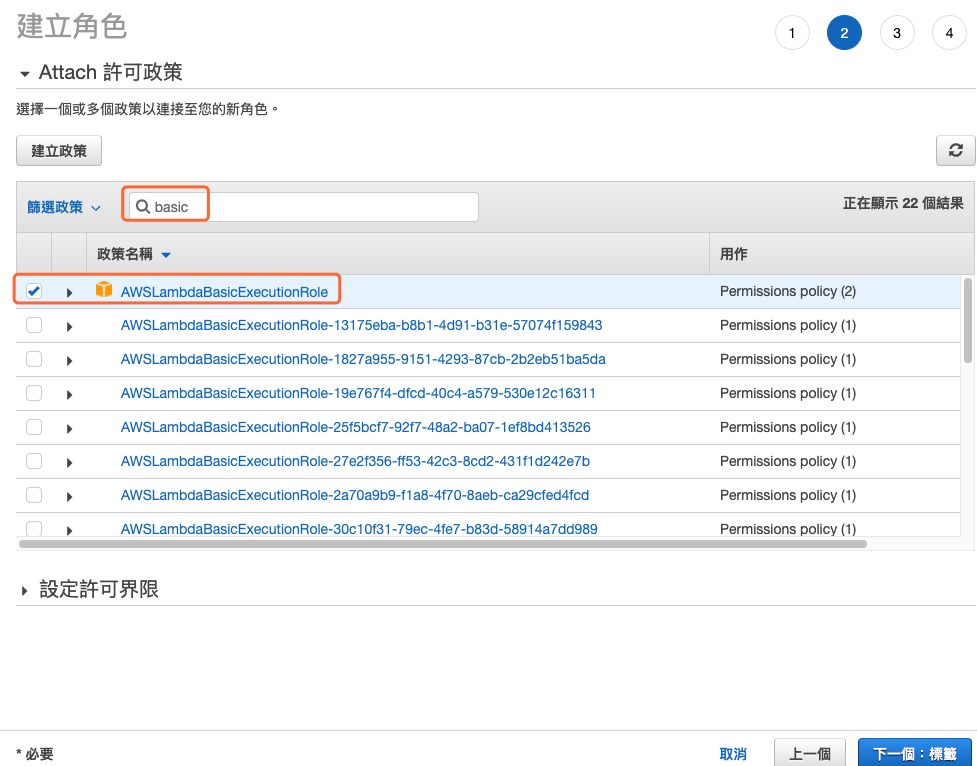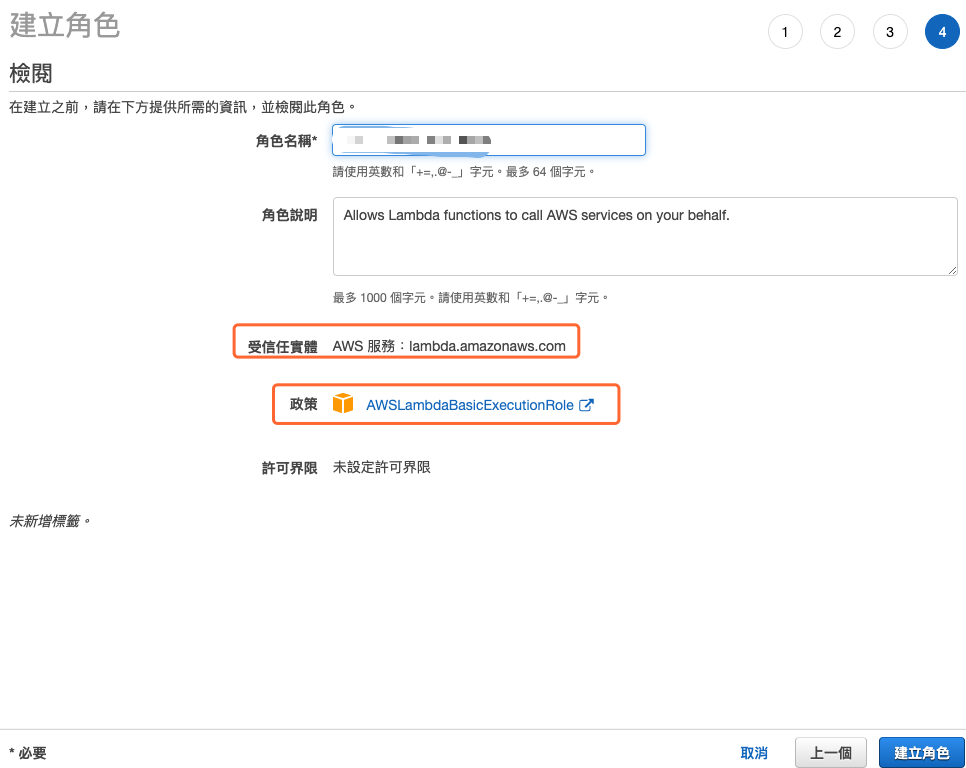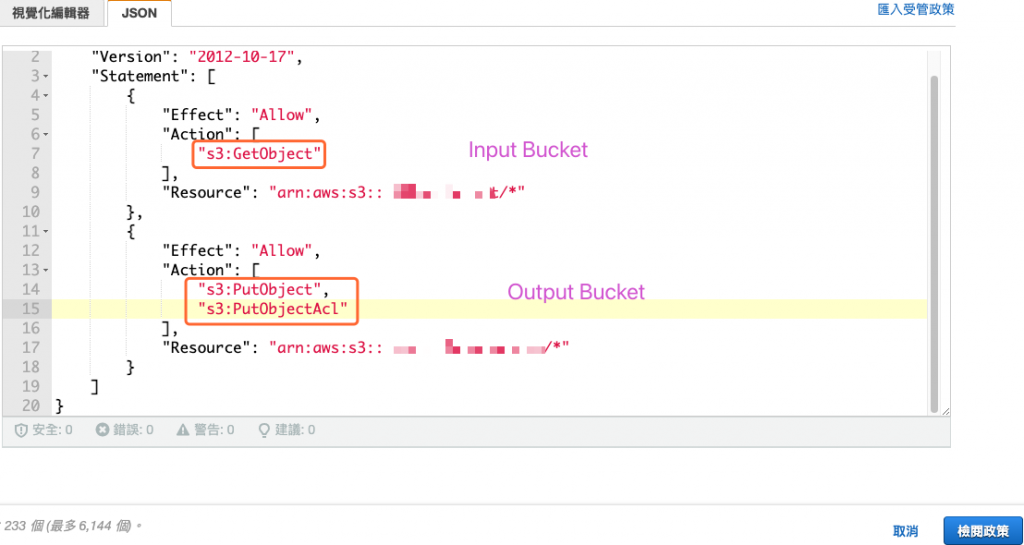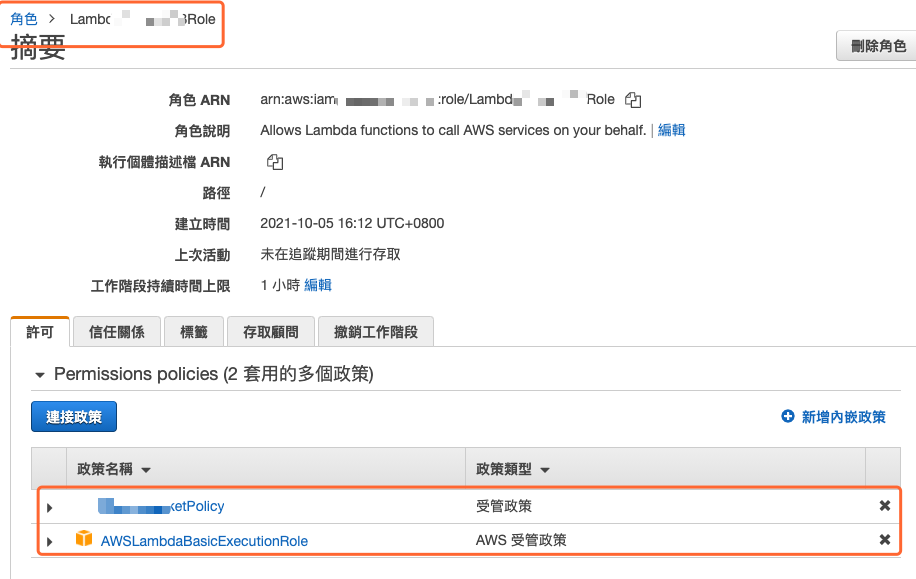• 選擇下列選項之一來建立您的函式： 從頭開始撰寫
• 函式名稱： opencv45Func
• 執行時間： Python 3.7
• 架構： x86_64
• 執行角色： 使用現有的角色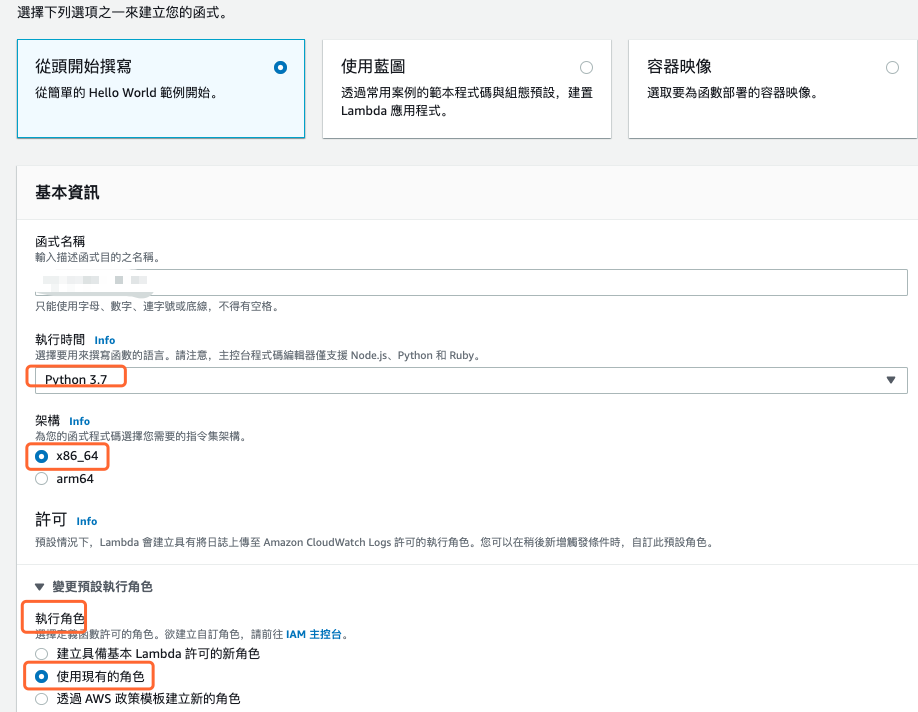• modelbucket： 存放權重的儲存貯體，應該也是儲存貯體 B，因為任何對 儲存貯體 A 所做的寫入都會引發驅動 Lambda 的事件。
• modelweight：yolov4cfg/yolov3.backup
• putbucket： 儲存貯體 B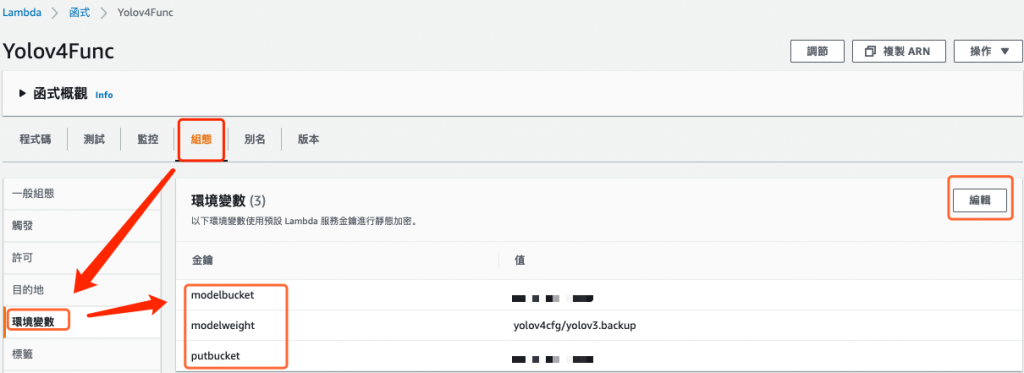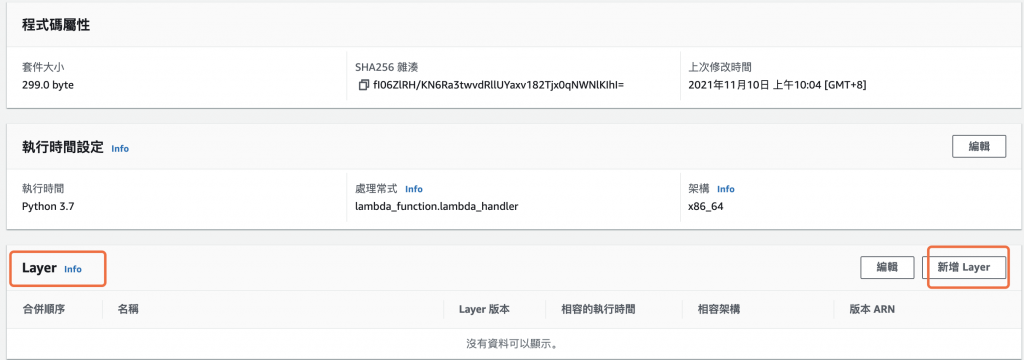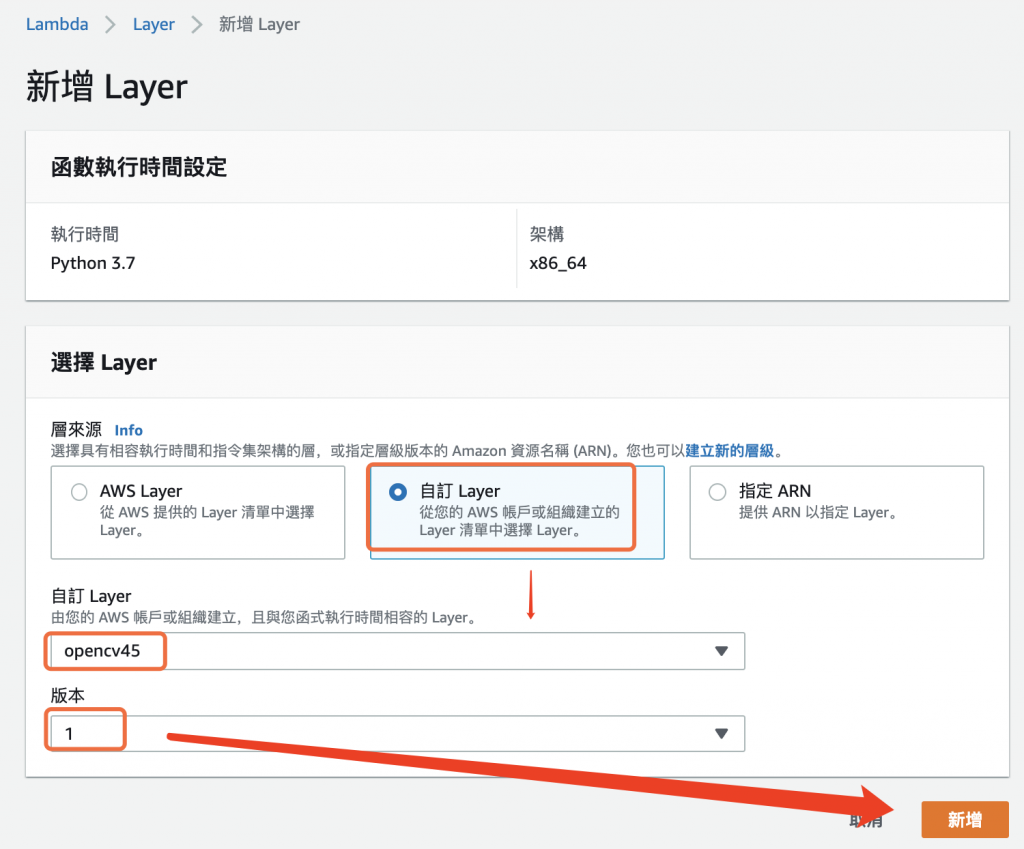service.py

``````from __future__ import print_function

import urllib.request
import os
import subprocess
import boto3
import time
import cv2

SCRIPT_DIR = os.path.dirname(os.path.abspath(__file__))
LIB_DIR = os.path.join(SCRIPT_DIR, 'lib')
s3_client = boto3.client('s3')
modelbucket = os.environ['modelbucket']
modelweight = os.environ['modelweight']
modelcfg = 'cfg/yolov3.cfg'

# 從 s3 下載檔案

strWeightFile = '/tmp/' +  modelweight.replace('/', '')
print(strWeightFile)

# 繪製物件方塊框
def draw_image(image_path, output_path, pridicts):
for k in range(len(pridicts)):
x, y, w, h  = pridicts[k]
cv2.putText(cv2image, "{:.4f}".format(pridicts[k]), (x, y-6), cv2.FONT_HERSHEY_SIMPLEX, 0.6, (0, 255, 0), 1, cv2.LINE_AA)
cv2.rectangle(cv2image, (int(x),int(y)), (int(x+w),int(y+w)), (0,255,0), 2)
cv2.imwrite(output_path, cv2image)

# yolo 推論函數
def yolo_infer(weight, cfg, pic):
net = cv2.dnn_DetectionModel(model)
net.setInputSize(608, 608)
net.setInputScale(1.0 / 255)
net.setInputSwapRB(True)

classes, confidences, boxes = net.detect(frame, confThreshold=0.1, nmsThreshold=0.4)
return classes,confidences,boxes

# lambda 程式進入口
def handler(event, context):
for record in event['Records']:
inputbucket = record['s3']['bucket']['name']
outputbucket = os.environ['putbucket']
key = record['s3']['object']['key']
imgfilepath = '/tmp/inputimage.jpg'

prev_time = time.time()
result = yolo_infer(strWeightFile, modelcfg, imgfilepath)
print('predicting time: ' , (time.time() - prev_time))

tmpkey = key.replace('/', '')

return 0
``````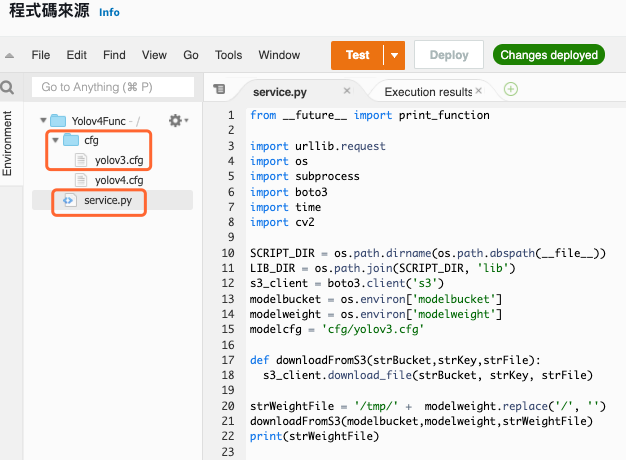``````{
"Records": [
{
"s3": {
"bucket": {
"name": "[INPUT_BUCKET]",
"arn": "arn:aws:s3:::[INPUT_BUCKET]"
},
"object": {
"key": "[INPUT_OBJECT]"
}
}
}
]
}
``````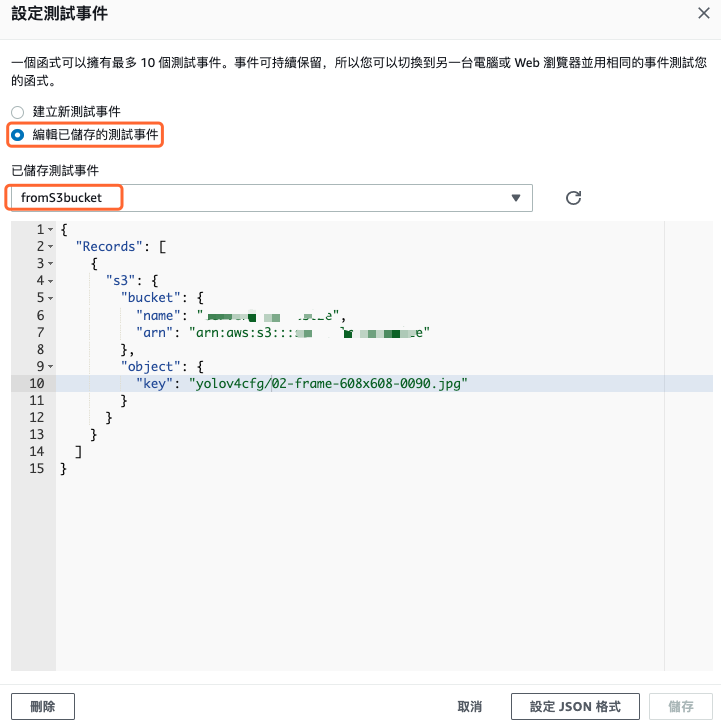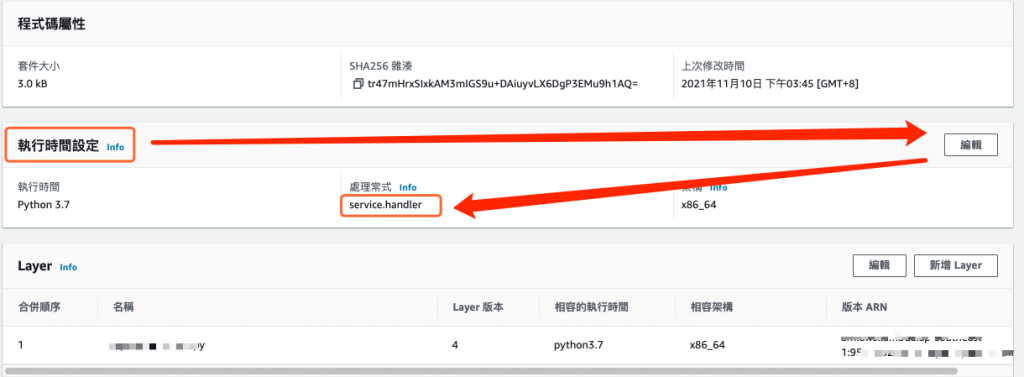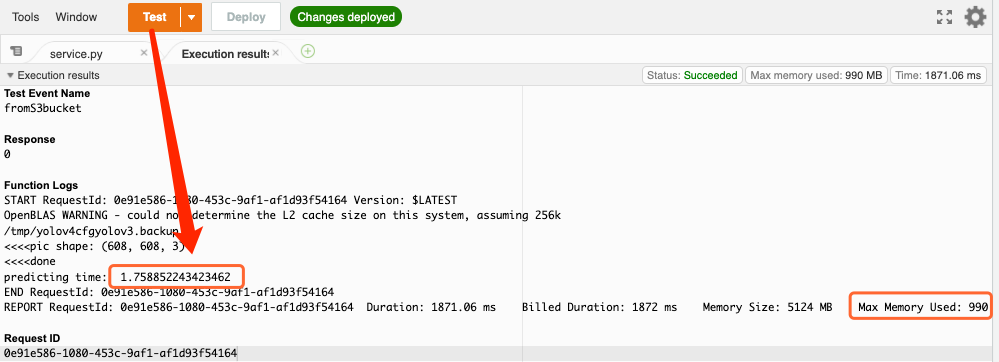使用 EC2 使用 Lambda (darknet) 使用 SageMaker 使用 Lambda (OpenCV)